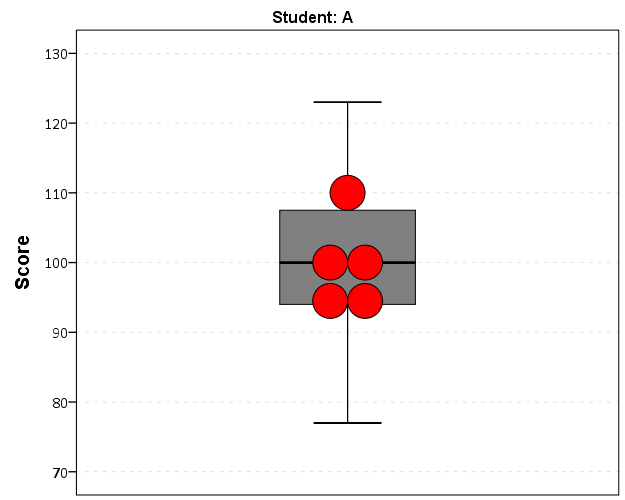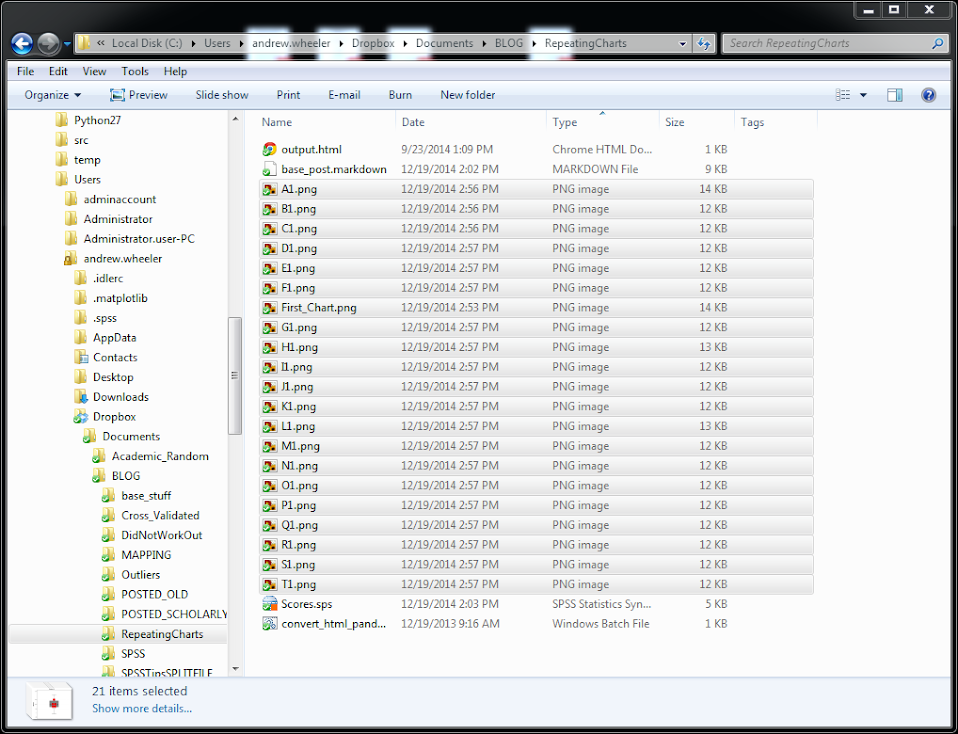# Repeating Charts in SPSS for unique IDs

The title is probably not that clear, but I’ve seen this request a few times and have used this trick in one my projects, so figured it would be a worthwhile topic to illustrate. So the problem is you have a background distribution, and you want to tailor a set of individual charts showing the unique individuals score against the background distribution. See two examples (1,2) of this question. In the first link I showed how one can do this by artificially duplicating the data in a specific way using `VARSTOCASES` and then using `SPLIT FILE` to generate the separate charts. Here I will show a python based solution that does not require duplicating the data.

So first we will start off with a set of fake student scores, 20 students with 5 scores each.

``````*Create some fake data, student test scores.
SET SEED 10.
INPUT PROGRAM.
STRING Student (A1).
LOOP #i = 1 TO 5.
LOOP #j = 1 TO 20.
COMPUTE Student = STRING(#j+64,PIB).
COMPUTE Score = RND(RV.NORMAL(100,10)).
END CASE.
END LOOP.
END LOOP.
END FILE.
END INPUT PROGRAM.
DATASET NAME Student_Scores.
FORMATS Score (F3.0).
EXECUTE.``````

Now the students are listed as strings, but here I am going to use `AUTORECODE` to automatically turn the strings into number variables, and more importantly for what follows create a set of value labels corresponding to those unique strings.

``````*Use Auto-recode to make the variables 1 to N.
AUTORECODE VARIABLES = Student /INTO Student_N.
FORMATS Student_N (F6.0).``````

Now the workflow I want to do is to make a set of charts with the background a boxplot for the whole class, and then the individual students scores as foreground dots. To do this I will make a second set of scores that are missing for everyone except that one particular student, the specify `MISSING=VARIABLEWISE` on the `GGRAPH` command, and then superimpose `Score2` over the boxplot of `Score`.

``````*Example of Individual chart.
NUMERIC flag (F1.0) Score2 (F3.0).

DO IF Student_N = 1.
COMPUTE Score2 = Score.
COMPUTE flag = 1.
ELSE.
COMPUTE Score2 = \$SYSMIS.
COMPUTE flag = 0.
END IF.

GGRAPH
/GRAPHDATASET NAME="graphdataset" VARIABLES=Score Score2 flag MISSING = VARIABLEWISE
/GRAPHSPEC SOURCE=INLINE.
BEGIN GPL
SOURCE: s=userSource(id("graphdataset"))
DATA: Score=col(source(s), name("Score"))
DATA: Score2=col(source(s), name("Score2"))
COORD: rect(dim(1), transpose())
GUIDE: axis(dim(1), label("Score"))
GUIDE: legend(aesthetic(aesthetic.visible), null())
GUIDE: text.title(label("Student: A"))
ELEMENT: schema(position(bin.quantile.letter(Score)), color.interior(color.grey))
ELEMENT: point.dodge.symmetric(position(bin.dot(Score2)), color.interior(color.red))
END GPL.
*cleaing up temp variables.
MATCH FILES FILE = * /DROP flag Score2.``````There are other ways to do this, like using the `visible` option in GPL aesthetics, but I don’t do that here because they still exist in the chart but simply aren’t shown. This causes problems with the dodging, and if you sent the chart in vector format the information would still be contained in the chart (e.g. if you need to aggregate the background data to be obfuscated for confidentiality reasons you don’t want it in the chart even if it is invisible). Here even the outlier dots in the boxplot are potentially disseminating confidential information, but for simplicity I don’t worry about that here (my syntax at the developerworks forum showed how you can build your own boxplot without having the points, it would be nice to have a no points option for the `schema` element).

So now the problem is looping through all of the individual students and generating a chart for each one. That is where the `AUTORECODE` comes in handy. I can grab all of the value labels from the SPSS dictionary and place them in a Python dictionary.

``````*Python to grab the different students.
BEGIN PROGRAM Python.
import spss
spss.StartDataStep()
datasetObj = spss.Dataset()
StudentLab = datasetObj.varlist['Student_N'].valueLabels
print StudentLab #this is a dictionary of all the unique students
spss.EndDataStep()
END PROGRAM.``````

Now with the `StudentLab` Python dictionary I can loop over the dictionary and submit the SPSS syntax for each unique student (using `spss.Submit`) using string substitutions. I first create the variables and set there formats outside of the loop (the chart inherits the formats). Then I just set several aesthetics of the charts so they are the same for every chart, e.g. the scale goes between 60 and 150, and the size of the superimposed points is 12.

``````*Now loop through the students.
OUTPUT CLOSE ALL.
BEGIN PROGRAM Python.
spss.Submit("NUMERIC flag (F1.0) Score2 (F3.0).")
for val, lab in StudentLab.data.iteritems():
spss.Submit("""*Individual score chart.
DO IF Student_N = %d.
COMPUTE Score2 = Score.
COMPUTE flag = 1.
ELSE.
COMPUTE Score2 = \$SYSMIS.
COMPUTE flag = 0.
END IF.
GGRAPH
/GRAPHDATASET NAME="graphdataset" VARIABLES=Score Score2 flag MISSING = VARIABLEWISE
/GRAPHSPEC SOURCE=INLINE.
BEGIN GPL
SOURCE: s=userSource(id("graphdataset"))
DATA: Score=col(source(s), name("Score"))
DATA: Score2=col(source(s), name("Score2"))
DATA: id=col(source(s), name("\$CASENUM"), unit.category())
DATA: flag=col(source(s), name("flag"), unit.category())
COORD: rect(dim(1), transpose())
GUIDE: axis(dim(1), label("Score"), delta(10), start(60))
GUIDE: text.title(label("Student: %s"))
GUIDE: legend(aesthetic(aesthetic.visible), null())
SCALE: linear(dim(1), min(60), max(150))
ELEMENT: schema(position(bin.quantile.letter(Score)), color.interior(color.grey))
ELEMENT: point.dodge.symmetric(position(bin.dot(Score2)), color.interior(color.red),
size(size."12"))
END GPL.
""" %(val,lab))
END PROGRAM.``````

I wanted to export these charts in the loop with the students names, using something like:

``````SpssOutputDoc.SelectLastOutput() #grab last output
SpssOutputDoc.ExportCharts(SpssClient.SpssExportSubset.SpssSelected, path + lab, SpssClient.ChartExportFormat.png)``````

but what happens with this is that it is always one behind (e.g. the first chart is selected in the second loop iteration). So what I did was to stuff all of the charts within a list and then loop over that list, select the chart, and then export it using `SpssOutputDoc.ExportCharts` (another option would be to use `EXPORT OUTPUT` and then either delete the output in-between charts or clear it, I wish OMS could export only charts). This would be more annoying with multiple individual charts, and could likely be made more concise, but here it is.

``````*Now exporting the individual charts.
BEGIN PROGRAM Python.
import SpssClient
SpssClient.StartClient()
SpssOutputDoc = SpssClient.GetDesignatedOutputDoc()

#creating a list of all the charts
OutputItems = SpssOutputDoc.GetOutputItems()
Charts = []
for index in range(OutputItems.Size()):
OutputItem = OutputItems.GetItemAt(index)
if OutputItem.GetType() == SpssClient.OutputItemType.CHART:
Charts.append(OutputItem)

labList = []
for val, lab in StudentLab.data.iteritems():
labList.append(lab)

path = "C:/Users/andrew.wheeler/Dropbox/Documents/BLOG/RepeatingCharts/"

for chart,lab in zip(Charts,labList):
SpssOutputDoc.ClearSelection() #clear prior selections
chart.SetSelected(True) #select chart
#export chart
SpssOutputDoc.ExportCharts(SpssClient.SpssExportSubset.SpssSelected, path + lab, SpssClient.ChartExportFormat.png)
END PROGRAM.``````

Here is a screen shot of the resulting images in my folder.So this will export the charts to PNG format with the image name the same as the students in the file (so the name needs to be in a format appropriate to save a file name). Annoyingly SPSS appends 1 to the end of all charts, even if it is only exporting one chart. Here is an example of the student G’s chart.Eventually I will figure out how to send emails via Python, and this would be a good tool for individualized report cards for a class. Here is a copy of the full syntax to more easily run on your local machine (just replace `path` with a location on your local machine).

1.#### Michael Rasmussen

/  December 28, 2014

Hi Andrew, Many thanks for this solution. This will make the work that I need to do a lot quicker. I’m a novice at SPSS and Python code, but your explanations are good, and I could find some definitions for the code on the web. I’d like to refer back to the original request
Given that the boxplots are distributed across each GRS, I was hoping you would be able to provide a little extra code that would suit multiple boxplots in the one plot. So Student A had a score for several different exams (3x observations for Student A). Are you able to handle that too?

•#### apwheele

/  December 28, 2014

Hi Mike, can you either send me a fake dataset that mimics yours (or update the SO post to include it.) What I have here is pretty close, you just need to update to your variable names and the base chart. (I will make sure to check in the SO post – but feel free to bug me with an email though if you get no response for a few days over at SO.)

2.#### Michael Rasmussen

/  December 29, 2014

Okay I will give it a try although I’m a little unsure as to how to paste a dataset into SO. Will give it a try anyhow. If it doesn’t work out, I will be sending an email through. Cheers Michael.

3.#### Jonas Steiner

/  November 1, 2016

Hey Michael
Have you managed to do it with multiple/clustered boxplot? Will you share your code? All the best, Jonas